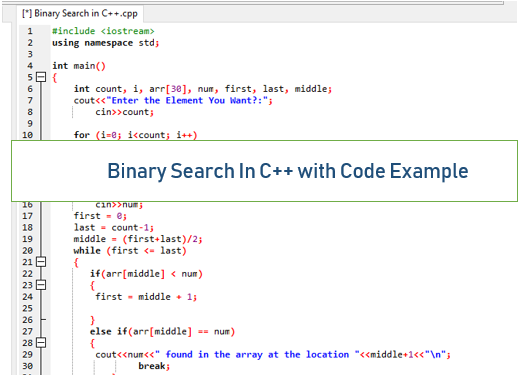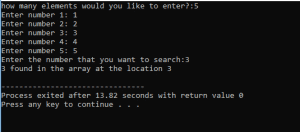# Binary Search in C++ with Code ExampleWritten by

What is Binary Search

Binary search is an effective algorithm for finding a item from a sorted list of things. It works by over and again dividing in half the portion of the list that could contain the item, until you’ve limited the potential areas to only one

Binary Search in c++ Code example

<iostream>
using namespace std;

int main()
{
int count, i, arr, num, first, last, middle;
cout<<“Enter the Element You Want?:”;
cin>>count;

for (i=0; i<count; i++)
{
cin>>arr[i];
}
cout<<“Enter the number that you are going to search:”;
cin>>num;
first = 0;
last = count-1;
middle = (first+last)/2;
while (first <= last)
{
if(arr[middle] < num)
{
first = middle + 1;

}
else if(arr[middle] == num)
{
cout<<num<<” found in the array at the location “<<middle+1<<“\n”;
break;
}
else {
last = middle – 1;
}
middle = (first + last)/2;
}
if(first > last)
{
}
return 0;
}

Out Put Screen